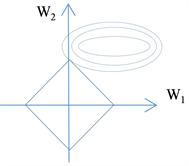# 从多重积分到Lasso——浅淡计算机科学与技术专业高等数学的教学From Multiple Integral to Lasso—A Note on Teaching of Advanced Mathematics Specified to the Department of Computer Science

• 全文下载: PDF(425KB)    PP.812-817   DOI: 10.12677/CES.2019.76138
• 下载量: 133  浏览量: 186

With the rapid development of machine learning, data science etc., many new problems about mathematics appeared in the class of computer science. Lasso is a key tool in solving optimization problems in machine learning, data science. In this paper, we will demonstrate Lasso in teaching of multiple integral and explore reforming the materials of advanced mathematics for the class of computer science.

1. 引言

Lasso是机器学习、大数据分析等当前研究的热点领域中非常重要的正则化工具。本文接下来以高等数学中的三重积分球面坐标积分法的教学为例，通过应用多重积分，阐述Lasso背后的数学原理，加深对该内容的理解，从而提高教学效果。

2. Lasso

${\text{min}}_{w}\underset{i=1}{\overset{n}{\sum }}{\left({y}_{i}-\underset{j=1}{\overset{d}{\sum }}{w}_{j}{x}_{ij}\right)}^{2}$,

${\mathrm{min}}_{w}\left[\underset{i=1}{\overset{n}{\sum }}{\left({y}_{i}-\underset{j=1}{\overset{d}{\sum }}{w}_{j}{x}_{ij}\right)}^{2}+\alpha |w|\right]$.

3. 多重积分与Lasso的数学原理

$\left\{\begin{array}{l}x=r\mathrm{cos}\phi \hfill \\ y=r\mathrm{sin}\phi \mathrm{cos}\theta \hfill \\ z=r\mathrm{sin}\phi \mathrm{sin}\theta \hfill \end{array}$,

${S}_{n}=\left\{\left({x}_{1},{x}_{2},\cdots ,{x}_{n}\right)|{x}_{1}^{2}+{x}_{2}^{2}+\cdots +{x}_{n}^{2}\le 1\right\}$

$\left\{\begin{array}{l}{x}_{1}=r\mathrm{cos}{\theta }_{1}\hfill \\ {x}_{2}=r\mathrm{sin}{\theta }_{1}\mathrm{cos}{\theta }_{2}\hfill \\ \text{\hspace{0.17em}}\text{\hspace{0.17em}}\text{\hspace{0.17em}}\text{\hspace{0.17em}}\text{\hspace{0.17em}}\text{\hspace{0.17em}}\text{\hspace{0.17em}}\text{\hspace{0.17em}}\text{\hspace{0.17em}}\text{\hspace{0.17em}}\text{\hspace{0.17em}}⋮\hfill \\ {x}_{n-1}=r\mathrm{sin}{\theta }_{1}\cdots \mathrm{sin}{\theta }_{n-1}\mathrm{cos}{\theta }_{n}\hfill \\ {x}_{n}=r\mathrm{sin}{\theta }_{1}\cdots \mathrm{sin}{\theta }_{n-1}\mathrm{sin}{\theta }_{n}\hfill \end{array}$,

$|J\left(n\right)|=|\begin{array}{cccc}\frac{\partial {x}_{1}}{\partial r}& \frac{\partial {x}_{1}}{\partial {\theta }_{1}}& \cdots & \frac{\partial {x}_{1}}{\partial {\theta }_{n-1}}\\ \frac{\partial {x}_{1}}{\partial r}& \frac{\partial {x}_{1}}{\partial {\theta }_{1}}& \cdots & \frac{\partial {x}_{1}}{\partial {\theta }_{n-1}}\\ ⋮& ⋮& \ddots & ⋮\\ \frac{\partial {x}_{1}}{\partial r}& \frac{\partial {x}_{1}}{\partial {\theta }_{1}}& \cdots & \frac{\partial {x}_{1}}{\partial {\theta }_{n-1}}\end{array}|={r}^{n-1}f\left({\theta }_{1},{\theta }_{2},\cdots ,{\theta }_{n-1}\right)$,

$V\left(n\right)=\underset{{x}_{1}=-1}{\overset{{x}_{1=1}}{\int }}\underset{{x}_{2}=-\sqrt{1-{x}_{1}^{2}}}{\overset{{x}_{2}=\sqrt{1-{x}_{1}^{2}}}{\int }}\cdots \underset{{x}_{n}=-\sqrt{1-{x}_{1}^{2}-\cdots -{x}_{n-1}^{2}}}{\overset{{x}_{n}=\sqrt{1-{x}_{1}^{2}-\cdots -{x}_{n-1}^{2}}}{\int }}\text{d}{x}_{n}\text{d}{x}_{n-1}\cdots \text{d}{x}_{1}$.

$V\left(n\right)={\int }_{\text{0}}^{\text{π}}\text{d}{\theta }_{\text{1}}{\int }_{0}^{\pi }\text{d}{\theta }_{2}\cdots {\int }_{0}^{\pi }\text{d}{\theta }_{n-2}{\int }_{0}^{2\pi }f\left({\theta }_{1},{\theta }_{2},\cdots ,{\theta }_{n-1}\right)\text{d}{\theta }_{n-1}{\int }_{0}^{1}{r}^{n-1}\text{d}r$.

$A\left(n\right)={\int }_{0}^{\pi }\text{d}{\theta }_{1}{\int }_{0}^{\pi }\text{d}{\theta }_{2}\cdots {\int }_{0}^{\pi }\text{d}{\theta }_{n-2}{\int }_{0}^{2\pi }f\left({\theta }_{1},{\theta }_{2},\cdots ,{\theta }_{n-1}\right)\text{d}{\theta }_{n-1}$,

$V\left(n\right)=\frac{1}{n}A\left(n\right)$.

$I\left(n\right)=\underset{-\infty }{\overset{+\infty }{\int }}\underset{-\infty }{\overset{+\infty }{\int }}\cdots \underset{-\infty }{\overset{+\infty }{\int }}{\text{e}}^{-\left({x}_{1}^{2}+{x}_{2}^{2}+\cdots +{x}_{n}^{2}\right)}\text{d}{x}_{n}\text{d}{x}_{n-1}\cdots \text{d}{x}_{1}$

$\left\{\begin{array}{l}{x}_{1}=r\mathrm{cos}{\theta }_{1}\hfill \\ {x}_{2}=r\mathrm{sin}{\theta }_{1}\mathrm{cos}{\theta }_{2}\hfill \\ \text{\hspace{0.17em}}\text{\hspace{0.17em}}\text{\hspace{0.17em}}\text{\hspace{0.17em}}\text{\hspace{0.17em}}\text{\hspace{0.17em}}\text{\hspace{0.17em}}\text{\hspace{0.17em}}\text{\hspace{0.17em}}\text{\hspace{0.17em}}\text{\hspace{0.17em}}⋮\hfill \\ {x}_{n-1}=r\mathrm{sin}{\theta }_{1}\cdots \mathrm{sin}{\theta }_{n-1}\mathrm{cos}{\theta }_{n}\hfill \\ {x}_{n}=r\mathrm{sin}{\theta }_{1}\cdots \mathrm{sin}{\theta }_{n-1}\mathrm{sin}{\theta }_{n}\hfill \end{array}$,

$I\left(n\right)={\int }_{0}^{\text{π}}\text{d}{\theta }_{1}{\int }_{0}^{\text{π}}\text{d}{\theta }_{2}\cdots {\int }_{0}^{\text{π}}\text{d}{\theta }_{n-2}{\int }_{0}^{2\text{π}}f\left({\theta }_{1},{\theta }_{2},\cdots ,{\theta }_{n-1}\right)\text{d}{\theta }_{n-1}{\int }_{0}^{+\infty }{r}^{n-1}\text{d}r=A\left(n\right){\int }_{0}^{+\infty }{\text{e}}^{-{r}^{2}}{r}^{n-1}\text{d}r$.

$\underset{0}{\overset{+\infty }{\int }}{\text{e}}^{-{r}^{2}}{r}^{n-1}\text{d}r=\frac{1}{2}\underset{0}{\overset{+\infty }{\int }}{\text{e}}^{-t}{t}^{\frac{n}{2}-1}\text{d}t=\frac{1}{2}\Gamma \left(\frac{n}{2}\right)$,

$V\left(n\right)=\frac{2{\pi }^{\frac{n}{2}}}{n\Gamma \left(\frac{n}{2}\right)}$,

$\underset{n\to +\infty }{\mathrm{lim}}\frac{2{\pi }^{\frac{n}{2}}}{n\Gamma \left(\frac{n}{2}\right)}=0$.

$\underset{n\to +\infty }{\mathrm{lim}}V\left(n\right)=0$.

${C}_{n}=\left\{\left({x}_{1},{x}_{2},\cdots ,{x}_{n}\right)||{x}_{1}|+|{x}_{2}|+\cdots +|{x}_{n}|\le 1\right\}$.

${\mathrm{min}}_{w}\left[\underset{i=1}{\overset{n}{\sum }}{\left({y}_{i}-\underset{j=1}{\overset{d}{\sum }}{w}_{j}{x}_{ij}\right)}^{2}+\alpha |w|\right]$

${\text{min}}_{w}\underset{i=1}{\overset{n}{\sum }}{\left({y}_{i}-\underset{j=1}{\overset{d}{\sum }}{w}_{j}{x}_{ij}\right)}^{2}$,

$|w|\le \lambda$

$|w|\le {\lambda }_{0}$ 相切时，此时对应的 $\left({w}_{1},{w}_{2},\cdots ,{w}_{d}\right)$ 即为满足不等约束的最优值点。以 $d=2$ 为例，我们用下面的图来表示这一过程4. 结语

  张春红. 浅析高等数学的教与学[J]. 科协论坛(下半月), 2013(8): 191-192.  黄加增. 浅谈高等数学在不同专业的教学改革与现实[J]. 数学学习与研究, 2011(23): 2-3.  张玲, 陈永强, 张华. 新工科建设背景下高等数学多目标教学模式探索[J]. 教书育人(高教论坛), 2019(9): 110-112.  远巧针. 高等数学教学中情感教育实施策略探讨[J]. 才智, 2019(9): 145-146.  李涛. 新建地方本科院校高等数学教学质量的评价研究[J]. 中国农村教育, 2019(5): 23-25.  程国, 余兴民. 应用型本科院校高等数学教学质量影响因素与对策研究[J]. 现代计算机(专业版), 2015(15): 23-27.